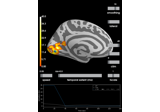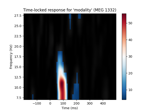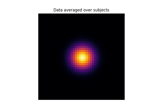# mne.stats.f_threshold_mway_rm¶

mne.stats.f_threshold_mway_rm(n_subjects, factor_levels, effects='A*B', pvalue=0.05)[source]

Compute F-value thresholds for a two-way ANOVA.

Parameters
n_subjectsint

The number of subjects to be analyzed.

factor_levelslist-like

The number of levels per factor.

effectsstr

A string denoting the effect to be returned. The following mapping is currently supported:

• 'A': main effect of A

• 'B': main effect of B

• 'A:B': interaction effect

• 'A+B': both main effects

• 'A*B': all three effects

pvaluefloat

The p-value to be thresholded.

Returns
F_threshold

List of F-values for each effect if the number of effects requested > 2, else float.

Notes

New in version 0.10.

## Examples using mne.stats.f_threshold_mway_rm¶